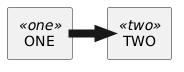How to change thickness of an arrow using <style>

This works

@startuml
rectangle one <<one>> as "ONE"
rectangle two <<two>> as "TWO"

one -[thickness=8]>> two
@endumlHowever as soon as I add style tags, the default style of the diagram changes and I lose the thickness of the arrow. But I don't know how to specify arrow thickness using the style tag

@startuml
<style>
{
}
</style>

rectangle one <<one>> as "ONE"
rectangle two <<two>> as "TWO"

one -[thickness=8]>> two
@endumlanswered Oct 2, 2021 by (37,800 points)

Hello D.,

For that you can use (but it is global and not local...):

@startuml
<style>
arrow {
LineThickness 9
}
</style>

rectangle one <<one>> as "ONE"
rectangle two <<two>> as "TWO"

one ->> two
@enduml